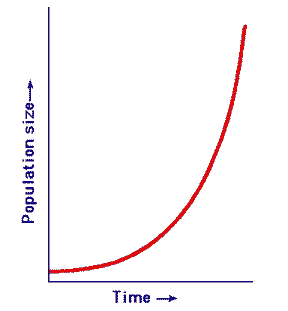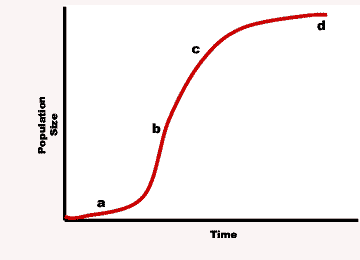Java Games: Flashcards, matching, concentration, and word search.# 2.11 Population Growth

AB
If you were told that there were 350 deer in an area that covers 10 square kilometers, how would you calculate the population density of the deer?You would divide the total population (in this case, 350 deer) by the area of land the population inhabits (in this case, 10 square kilometers) to get the population density (in this case, 35 deer per square kilometer)
The movement of organisms into a given area from another area is called ____.immigration
When organisms leave a certain area, the movement is called ___.emigration
For a population to grow, the ____ rate must be bigger than the ____ rate (assuming no migration into or out of the area).birthrate must be larger than the deathrate
When individuals reproduce at a constant rate, ____ population growth occurs.exponential (For example, bacteria dividing at a constant rate of once every 20 minutes can create a colony of over a million bacteria from one original bacterium in less than 7 hours),The various growth phases through which most populations go are represented on a(an) ____.logistic growth curve,As resources in a population become less available, the population will ___.reach its carrying capacity
In a logistic growth curve, exponential growth is the phase in which the population ___.grows more and more rapidly
When the exponential phase of a logistic growth curve of a population ends, the ____.population continues to grow, but at a slower and slower rate.
The number of individuals per unit area is a population’s ____.density
Logistic growth occurs when a population’s growth rate starts to __________ , after a period of rapid growth.slows down
Under conditions of logistic growth, population size will rise and fall around an average point called the ____.carrying capacity
Under ideal conditions with unlimited resources, a population will grow _____.exponentially
Which type of population growth does this graph show?,Exponential growth (which only happens under ideal conditions with unlimited space, food and no predators),Which type of population growth does this graph show?,Logistic growth,At which point in the graph below is the population growth rate accelerating?,Point A,At which point in the graph below is the population growth rate the highest?,The population growth "rate" is the highest at point B (Notice that the slope at point B is the steepest. The slope, rise over run, equals population growth/rise divided by time/run, which equals the population growth "rate"),At which point in the graph below is the population growth rate slowing down?,Point C,At which point in the graph below is the population the highest?,The population is the highest at point D (look along the y-axis),At which point on the graph below is the population growth rate the lowest?,The population growth rate is lowest at point D (notice that the population, even though it is at its highest, has stopped growing),At which point in the graph below is the population at the carrying capacity?,The population has reached the carrying capacity at point D (Remember, the carrying capacity is the maximum number of individuals that the environment can support),Limiting factors are factors that cause the _______ to decrease.population growth rate
What do we call factors that cause population growth rates to decrease?Limiting factors
Give five examples of limiting factors.Competition, predation, parasitism and disease, unnatural climate extremes, human disturbances
Give 2 examples of unnatural climate extremes that can act as limiting factors.Drought and ice ages
Give two examples of human disturbances that can act as limiting factors.Deforestation and oil spills
What is a density-dependent limiting factor?A density-dependent limiting factor is a limiting factor that affects denser populations more than less dense populations.
What is a density-independent limiting factor?A density-independent factor is a limiting factor that affects dense populations just as much as less dense populations.
Give 3 examples of density-dependent limiting factors.Competition, predation, parasitism and disease
Give 2 examples of density-independent factors.Unnatural climate extremes and human disturbancesThis activity was created by a Quia Web subscriber. Learn more about QuiaCreate your own activities